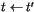Next: OpenGL Speedup Up: Contetns Previous: State of the Art

Subsections

# 3 SGI-based Algorithm Implementation

In this section some details about SGI-based stripification algorithm will be explained. The SGI tomesh was developed for generic triangle strips. Because it was designed for GL which supports a swap command, it doesn't try to avoid the swaps. This is no problem for the GL library because you need to send only one bit per swap. But for OpenGL library it is one vertex per swap. This means that the generalized strip containing n triangles could be encoded with more than n+2 vertices.

## 3.1 Basic Algorithm

The basic algorithm consists of several steps:
1. If there are no more triangles in the triangulation then exit.
2. Find the triangle t with the least number of neighbors (if more than one exists, choose arbitrary).
3. Start a new strip.
4. Insert the triangle t to the strip and remove it from the triangulation.
5. If there is no neighboring triangle to triangle t then go to 1.
6. Choose a new triangle t', neighboring to triangle t, with the least number of neighbors. If there is more than one triangle t' with the same least number of neighbors, look one level ahead. If there is a tie again, choose t' arbitrarily.
7.. Go to 4.

The effectiveness of the second step is crucial for the algorithm complexity. The best possible complexity is a linear function of the number of triangles n . If the first step is implemented as a sequential search then the algorithm complexity is close to O(n.s), where s is the number of triangle strips. To avoid this growth of complexity, some extra-structures have to be used (lists, priority qeue, etc.).

## 3.2 Choosing next triangle

Because step 6 - i.e., choosing next triangle - is a heuristic and has the most significant impact on the strip quality, we decided to try some other heuristic functions and study the influence on the results. We have tested these heuristics:

1. Choose the next triangle randomly (thereinafter RN).
2. Choose the triangle with the least number of neighbors.
If more than one exists, choose arbitrary (thereinafter LNRN).
3. Choose the triangle with the least number of neighbors.
If more than one exists then look one level ahead (original heuristic - thereinafter LNLN).
4. Choose the triangle with the least number of neighbors.
If more than one exists then choose the one that does not produce a swap (thereinafter LNLS).

The first heuristic is very easy to implement. It should be fast, but it does not reflect the main idea of the algorithm - not to break the existing triangulation into many small parts. The second heuristic is more complex than the first one, but still more simple than the original one. The result should be better (less fragmented triangulation) than in the first case. The last heuristic is about the same complexity as the original (third) one. Instead of trying to look ahead when a tie occurs, a test for a swap is made. This heuristic should give more strips than the original one, but probably less vertices.

Next: OpenGL Speedup Up: Contetns Previous: State of the Art

Petr Vanecek, pet@students.zcu.cz, 2002-03-19